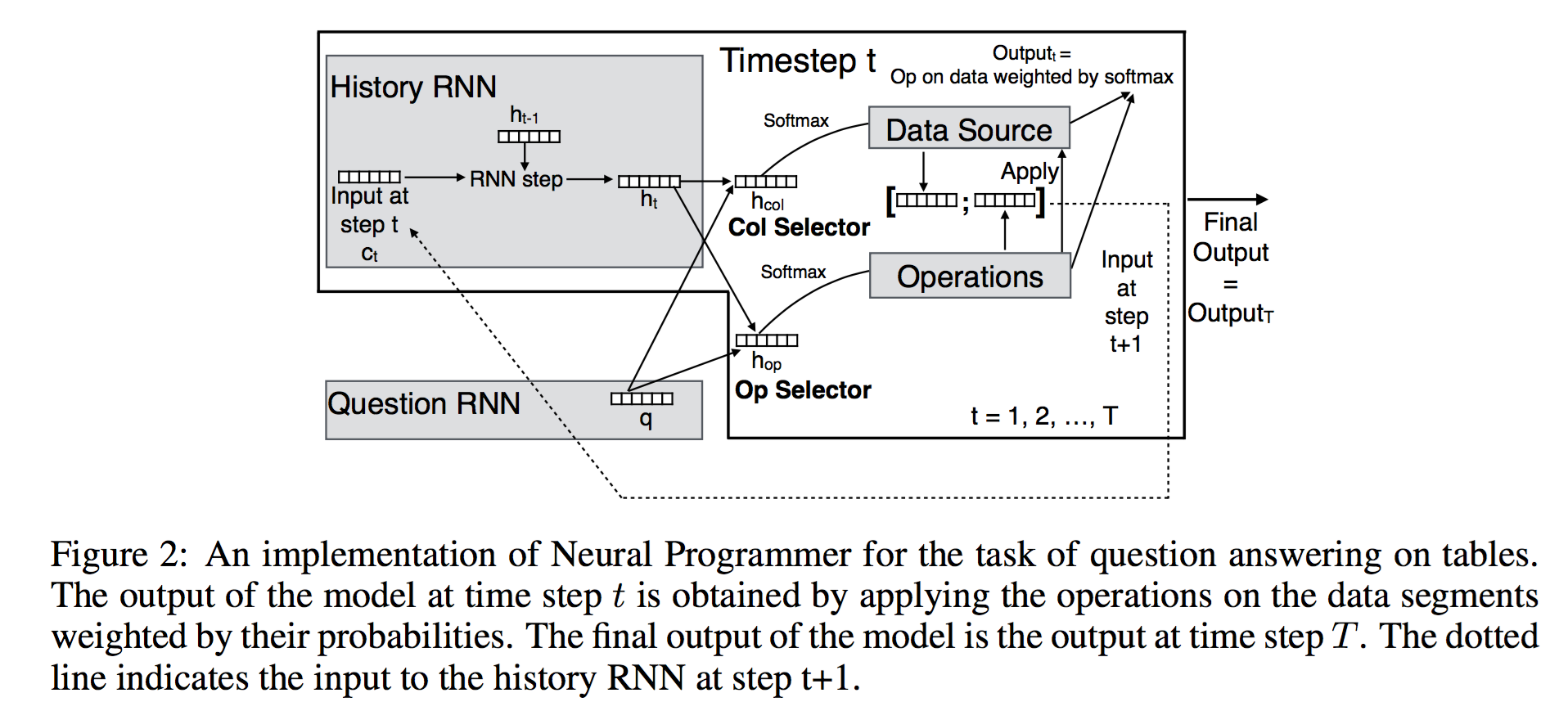## 关于

1. Neural Programmer: Inducing Latent Programs with Gradient Descent, Arvind Neelakantan, Quoc V Le, Ilya Sutskever, ICLR 2016

## 摘要

A major limitation of these models is in their inability to learn even simple arithmetic and logic operations.
recurrent neural networks (RNNs) fail at the task of adding two binary numbers even when the result has less than 10 bits

## 模型

Neural Programmer 由3个部分构成：

1. question Recurrent Neural Network (RNN) 处理用自然语言输入的问题
2. selector 生成两个概率分布，用于（soft select）选择数据分片和操作
3. history RNN 记住历史选择的数据分片和操作data source 以表格形式存在 $table \in \mathbb{R}^{M \times C}$

QUESTION MODULE 是一个简单的 RNN 模块，将输入的词序列（分布是表达）编码成一个 d 维的向量q。

SELECTOR 生成两个分布，一个是operator的概率分布，一个是数据列的概率分布（问题：数据列是变动的，怎么办？）

$$\alpha_t^{op} = softmax(U tanh(W^{op} [q; h_t]))$$

$$\alpha_t^{col} = softmax(P tanh(W^{col} [q; h_t]))$$

$$\beta_{op} = softmax(Z U(op)) \\ pivot_{op} = \sum_{i=1}^N \beta(i) qn_i$$